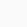# How to use floor symbol ⌊..⌋ in LaTeX?

Symbol/UnicodeFloor
Type of symbolMathematics
Package (requirement)No
ArgumentYes
Latex command\lfloor arg \rfloor
Example\lfloor arg \rfloor → ⌊arg⌋

You need to use the \lfloor arg \rfloor command to print the floor symbol in a latex document. But if the equation is large, I would recommend using the \left\lfloor arg \right\rfloor command. By this, the size of the symbol will be automatically adjusted as the size of the equation.

\documentclass{article}
\begin{document}
$\lfloor a, b \rfloor$
$\left\lfloor \frac{2m+x}{n} \right\rfloor$
$\left\lfloor \frac{(n-1)m+x}{n} \right\rfloor$
\end{document}

Output :Scroll to Top